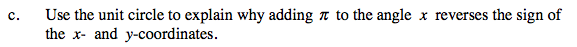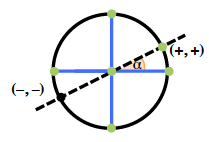### Home > PC > Chapter 4 > Lesson 4.1.4 > Problem4-57

4-57.
1. Consider the graphs of y = sin(x +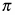) and y = −sin x. Homework Help ✎

1. Sketch both graphs on separate axes. What do you notice?

2. Sketch the graph of y = cos(x +). What is it equivalent to?

3. Use the unit circle to explain why addingto the angle x reverses the sign of the x- and y-coordinates.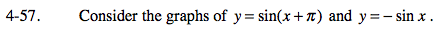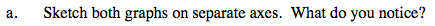The first curve is shifted left π units.
The second curve is reflected vertically.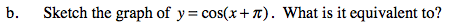Look at the equations of the curves and the hint in part (a).